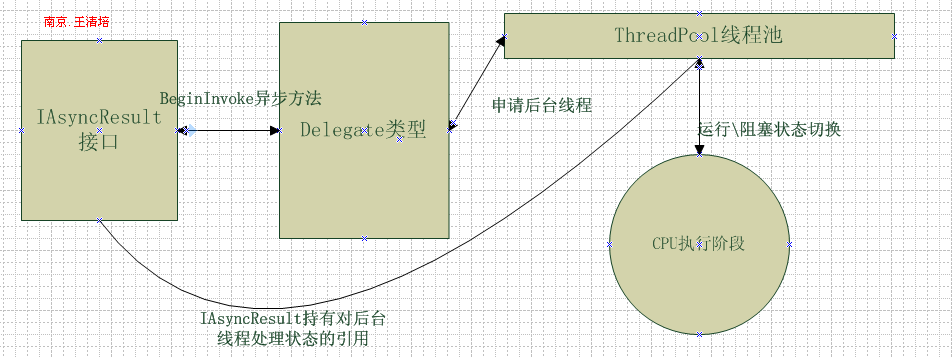.NET简谈组件程序设计之(异步委托)

0
0
01. 云栖社区>
2. 博客>
3. 正文

.NET简谈组件程序设计之(异步委托)

说到委托我想大家基本上都用过的，今天这篇文章就来讲解关于委托的异步奥秘。

using System;
using System.Collections.Generic;
using System.Text;
using System.Runtime;

namespace ConsoleApplication1
{
/// <summary>
/// 用来计算的委托
/// </summary>
/// <param name="i">参数一</param>
/// <param name="y">参数二</param>
/// <returns>计算的结果</returns>
public delegate int BinaryOperaton(int i, int y);
/// <summary>
/// 计算类，隔离上下文
/// </summary>
public class Calculator : ContextBoundObject
{
/// <summary>
/// 两数相加
/// </summary>
/// <param name="argument1"></param>
/// <param name="argument2"></param>
/// <returns></returns>
public int Add(int argument1, int argument2)
{
return argument1 + argument2;
}
/// <summary>
/// 两数相减
/// </summary>
/// <param name="argument1"></param>
/// <param name="argument2"></param>
/// <returns></returns>
public int Subtract(int argument1, int argument2)
{
return argument1 - argument2;
}
}
}

这是一段普通的代码，包含一个委托定义、一个计算类。等一下我们就用这个定义的委托来讲解委托的异步调用。。[王清培版权所有，转载请给出署名]

//同步调用
Calculator calcularor = new Calculator();
BinaryOperaton operation = calcularor.Add;
Console.WriteLine(operation(10, 20));

Calculator calcularor = new Calculator();
BinaryOperaton operation = calcularor.Add;
IAsyncResult result = operation.BeginInvoke(10, 20, null, null);//异步委托只能有一个方法的引用  Console.ReadLine();//AsyncResult中的AsyncDelegate
Calculator calcularor = new Calculator();
BinaryOperaton operation = calcularor.Add;
//IAsyncResult接口在委托使用中返回的强类型是AsyncResult委托异步状态对象
AsyncResult result = (AsyncResult)operation.BeginInvoke(10, 20, null, null);
if (!result.EndInvokeCalled)
{
//通过AsyncResult可以获取到当初调用BeginInvoke的委托实例
int count = (result.AsyncDelegate as BinaryOperaton).EndInvoke(result);
Console.WriteLine(count);
}

//异步调用.循环等待
Calculator calcularor = new Calculator();
BinaryOperaton operation = calcularor.Add;
IAsyncResult result = operation.BeginInvoke(10, 20, null, null);//异步委托只能有一个方法的引用
while (!result.IsCompleted)//通过IsCompleted进行判断异步操作是否已经结束,true已经结束,false未结束
{
int count = operation.EndInvoke(result);
Console.WriteLine(count);
}

//轮询等待完成
Calculator calcularor = new Calculator();
BinaryOperaton operation = calcularor.Add;
IAsyncResult result = (AsyncResult)operation.BeginInvoke(10, 20, null, null);
//阻塞当前线程直到方法结束，等待4秒钟。如果当前实例收到信号返回true,否则false.
while (true)
{
if (result.AsyncWaitHandle.WaitOne(4000))
break;
}
int count = operation.EndInvoke(result);
Console.WriteLine(count);

//异步回调
Calculator calcularor = new Calculator();
BinaryOperaton operation = calcularor.Add;
operation.BeginInvoke(10, 20, OnCompletion, true);//异步回调可以传入适当的参数

static void OnCompletion(IAsyncResult asyresult)
{
AsyncResult comresult = asyresult as AsyncResult;
int count = (comresult.AsyncDelegate as BinaryOperaton).EndInvoke(comresult);
if ((bool)comresult.AsyncState == true)
Console.WriteLine("true");
Console.WriteLine(count);

}

//异步事件
Delegate[] delegatelist = numberchangle.GetInvocationList();
foreach (Delegate dele in delegatelist)//异步事件必须分开调用
{
(dele as BinaryOperaton).BeginInvoke(10, 20, null, null);
}

+ 关注

corcosa 15110人浏览